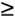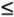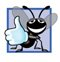# Decision Making: Equality and Relational Operators

### Decision Making Equality and Relational Operators

A condition is an expression that can be either true or false. This section introduces a simple version of Java's if statement that allows a program to make a decision based on the value of a condition. For example, the condition "grade is greater than or equal to 60" determines whether a student passed a test. If the condition in an if statement is true, the body of the if statement executes. If the condition is false, the body does not execute. We will see an example shortly.

Conditions in if statements can be formed by using the equality operators (== and !=) and relational operators (>, <, >= and <=) summarized in Fig. 2.14. Both equality operators have the same level of precedence, which is lower than that of the relational operators. The equality operators associate from left to right. The relational operators all have the same level of precedence and also associate from left to right.

Figure 2.14. Equality and relational operators.

Standard algebraic equality or relational operator

Java equality or relational operator

Sample Java condition

Meaning of Java condition

Equality operators

=

==

x == y

x is equal to y!=

x != y

x is not equal to y

Relational operators

>

>

x > y

x is greater than y

<

<

x < y

x is less than y>=

x >= y

x is greater than or equal to y<=

x <= y

x is less than or equal to y

The application of Fig. 2.15 uses six if statements to compare two integers input by the user. If the condition in any of these if statements is true, the assignment statement associated with that if statement executes. The program uses a Scanner to input the two integers from the user and store them in variables number1 and number2. Then the program compares the numbers and displays the results of the comparisons that are true.

Figure 2.15. Equality and relational operators.

(This item is displayed on pages 57 - 58 in the print version)

``` 1 // Fig. 2.15: Comparison.java
2 // Compare integers using if statements, relational operators
3 // and equality operators.
4 import java.util.Scanner; // program uses class Scanner
5
6 public class Comparison
7 {
8 // main method begins execution of Java application
9 public static void main( String args[] )
10 {
11 // create Scanner to obtain input from command window
12 Scanner input = new Scanner( System.in );
13
14 int number1; // first number to compare
15 int number2; // second number to compare
16
17 System.out.print( "Enter first integer: " ); // prompt
18 number1 = input.nextInt(); // read first number from user
19
20 System.out.print( "Enter second integer: " ); // prompt
21 number2 = input.nextInt(); // read second number from user
22
23 if ( number1 == number2 )
24  System.out.printf( "%d == %d
", number1, number2 );
25
26 if ( number1 != number2 )
27  System.out.printf( "%d != %d
", number1, number2 );
28
29 if ( number1 < number2 )
30  System.out.printf( "%d < %d
", number1, number2 );
31
32 if ( number1 > number2 )
33  System.out.printf( "%d > %d
", number1, number2 );
34
35 if ( number1 <= number2 )
36  System.out.printf( "%d <= %d
", number1, number2 );
37
38 if ( number1 >= number2 )
39  System.out.printf( "%d >= %d
", number1, number2 );
40
41 } // end method main
42
43 } // end class Comparison
```

 ```Enter first integer: 777 Enter second integer: 777 777 == 777 777 <= 777 777 >= 777 ```

 ```Enter first integer: 1000 Enter second integer: 2000 1000 != 2000 1000 < 2000 1000 <= 2000 ```

 ```Enter first integer: 2000 Enter second integer: 1000 2000 != 1000 2000 > 1000 2000 >= 1000 ```

The declaration of class Comparison begins at line 6

` public class Comparison`

The class's main method (lines 941) begins the execution of the program.

Line 12

``` Scanner input = new Scanner( System.in );
```

declares Scanner variable input and assigns it a Scanner that inputs data from the standard input (i.e., the keyboard).

Lines 1415

``` int number1; // first number to compare
int number2; // second number to compare
```

declare the int variables used to store the values input from the user.

Lines 1718

``` System.out.print( "Enter first integer: " ); // prompt
number1 = input.nextInt(); // read first number from user
```

prompt the user to enter the first integer and input the value, respectively. The input value is stored in variable number1.

Lines 2021

``` System.out.print( "Enter second integer: " ); // prompt
number2 = input.nextInt(); // read second number from user
```

prompt the user to enter the second integer and input the value, respectively. The input value is stored in variable number2.

Lines 2324

``` if ( number1 == number2 )
System.out.printf( "%d == %d
", number1, number2 );
```

declare an if statement that compares the values of the variables number1 and number2 to determine whether they are equal. An if statement always begins with keyword if, followed by a condition in parentheses. An if statement expects one statement in its body. The indentation of the body statement shown here is not required, but it improves the program's readability by emphasizing that the statement in line 24 is part of the if statement that begins on line 23. Line 24 executes only if the numbers stored in variables number1 and number2 are equal (i.e., the condition is true). The if statements at lines 2627, 2930, 3233, 3536 and 3839 compare number1 and number2 with the operators !=, <, >, <= and >=, respectively. If the condition in any of the if statements is true, the corresponding body statement executes.

Common Programming Error 2.9Forgetting the left and/or right parentheses for the condition in an if statement is a syntax errorthe parentheses are required.

Common Programming Error 2.10Confusing the equality operator, ==, with the assignment operator, =, can cause a logic error or a syntax error. The equality operator should be read as "is equal to," and the assignment operator should be read as "gets" or "gets the value of." To avoid confusion, some people read the equality operator as "double equals" or "equals equals."

Common Programming Error 2.11It is a syntax error if the operators ==, !=, >= and <= contain spaces between their symbols, as in = =, ! =, > = and < =, respectively.

Common Programming Error 2.12Reversing the operators !=, >= and <=, as in =!, => and =<, is a syntax error.

Good Programming Practice 2.15Indent an if statement's body to make it stand out and to enhance program readability.

Good Programming Practice 2.16Place only one statement per line in a program. This format enhances program readability.

Note that there is no semicolon (;) at the end of the first line of each if statement. Such a semicolon would result in a logic error at execution time. For example,

``` if ( number1 == number2 ); // logic error
System.out.printf( "%d == %d
", number1, number2 );
```

would actually be interpreted by Java as

``` if ( number1 == number2 )
; // empty statement

System.out.printf( "%d == %d
", number1, number2 );
```

where the semicolon on the line by itselfcalled the empty statementis the statement to execute if the condition in the if statement is true. When the empty statement executes, no task is performed in the program. The program then continues with the output statement, which always executes, regardless of whether the condition is true or false, because the output statement is not part of the if statement.

Common Programming Error 2.13Placing a semicolon immediately after the right parenthesis of the condition in an if statement is normally a logic error.

Note the use of white space in Fig. 2.15. Recall that white-space characters, such as tabs, newlines and spaces, are normally ignored by the compiler. So statements may be split over several lines and may be spaced according to the programmer's preferences without affecting the meaning of a program. It is incorrect to split identifiers and strings. Ideally, statements should be kept small, but this is not always possible.

Good Programming Practice 2.17A lengthy statement can be spread over several lines. If a single statement must be split across lines, choose breaking points that make sense, such as after a comma in a comma-separated list, or after an operator in a lengthy expression. If a statement is split across two or more lines, indent all subsequent lines until the end of the statement.

Figure 2.16 shows the precedence of the operators introduced in this chapter. The operators are shown from top to bottom in decreasing order of precedence. All these operators, with the exception of the assignment operator, =, associate from left to right. Addition is left associative, so an expression like x + y + z is evaluated as if it had been written as ( x + y ) + z. The assignment operator, =, associates from right to left, so an expression like x = y = 0 is evaluated as if it had been written as x = ( y = 0 ), which, as we will soon see, first assigns the value 0 to variable y and then assigns the result of that assignment, 0, to x.

Figure 2.16. Precedence and associativity of operations discussed.

Operators

Associativity

Type

*

/

%

left to right

multiplicative

+

-

left to right

<

<=

>

>=

left to right

relational

==

!=

left to right

equality

=

right to left

assignment

Good Programming Practice 2.18Refer to the operator precedence chart (see the complete chart in Appendix A) when writing expressions containing many operators. Confirm that the operations in the expression are performed in the order you expect. If you are uncertain about the order of evaluation in a complex expression, use parentheses to force the order, exactly as you would do in algebraic expressions. Observe that some operators, such as assignment, =, associate from right to left rather than from left to right.Java How to Program (6th Edition) (How to Program (Deitel))
ISBN: 0131483986
EAN: 2147483647
Year: 2003
Pages: 615